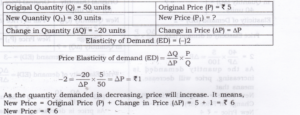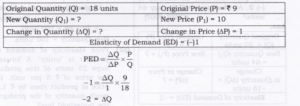# Chapter 4 – Elasticity of Demand Questions and Answers: NCERT Solutions for Class 12 Micro Economics

Class 12 Introduction to Economics NCERT book solutions for Chapter 4 - Elasticity of Demand Questions and Answers.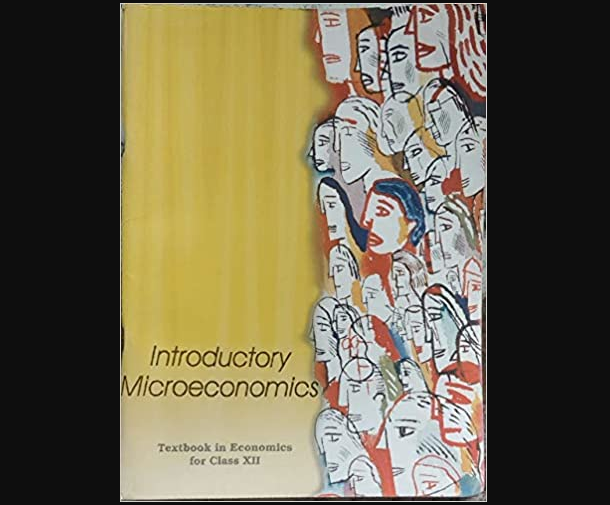## Question 2. Consider the demand for a good. At price Rs 4, the demand for the good is 25 units. Suppose price of the good increases to Rs 5, and as a result, the demand for the good falls to 20 units. Calculate the price elasticity? [3-4 Marks]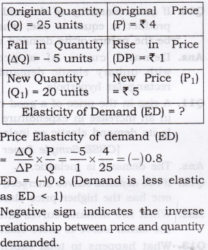## Question 3.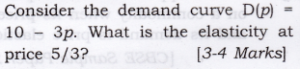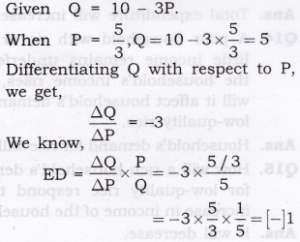## Question 4. Suppose the price elasticity of demand for a good is -0.2. If there is a 5% increase in the price of the good, by what percentage will the demand for the good go down?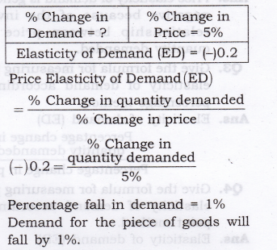## Question 1. Differentiate between perfectly elastic and perfectly inelastic demand.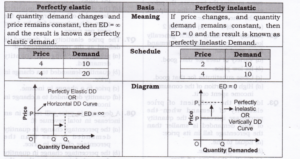## Question 2. When price is Rs. 20 per unit, demand for a commodity is 500 units. As the price falls to Rs. 15 per unit, demand expands to 800 units. Calculate elasticity of demand.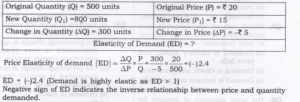## Question 3. The demand for a goods falls to 500 units in response to rise in price by Rs. 10. If the original demand was 600 units at the price of Rs. 30, calculate price elasticity of demand.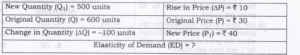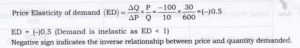## Question 4. A consumer spends Rs. 80 on a commodity when price is Rs. 1 per unit. If the price increases by Rs. 1, his expenditure becomes Rs. 96. Comment on PED.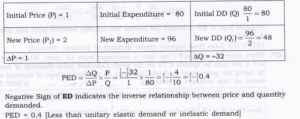## Question 5. A decline in the price of good X by Rs. 5 causes an increase in its demand by 20 units to 50 units. The new price is X 15. Calculate elasticity of demand.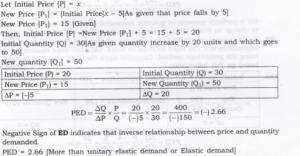## Question 6. A dentist was charging Rs. 300 for a standard cleaning job, and per month it used to generate total revenue equal to Rs. 30,000. She has increased the price of dental cleaning to Rs. 350 since last month. As the result of, few customers are now coming for dental clearing, but the total revenue is now Rs. 33,250. From this, what can we conclude about the elasticity of demand for such a dental service. Calculate PED by proportionate method.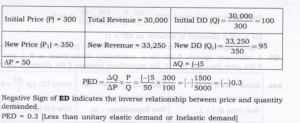## Question 7. Negative Sign of ED indicates the inverse relationship between price and quantity demanded. PED = 0.3 [Less than unitary elastic demand or Inelastic demand] When price of a good is Rs. 7 per unit, a consumer buys 12 units. When price falls to Rs. 6 per unit he spends Rs. 72 on the good. Calculate price elasticity of demand by using the percentage method. Comment on the likely shape of demand curve based on this measure of elasticity.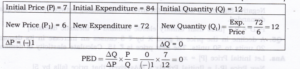## Question 8. A consumer buys 20 units of a good at a price of Rs. 5 per unit. He incurs an expenditure of Rs. 120 when he buys 24 units. Calculate price elasticity of demand using the percentage method. Comment upon the likely shape of demand curve based on this information.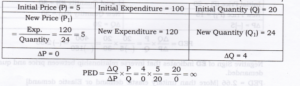## Question 9. A consumer buys 10 units of a commodity at a price of Rs. 10 per unit. He incurs an expenditure of Rs. 200 on buying 20 units. Calculate price elasticity of demand by the percentage method. Comment upon the shape of demand curve based on this information.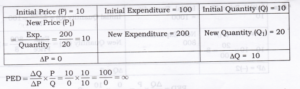## Question 10. ED is perfectly inelastic as quantity demanded does not change at all in response to change in price. Thus, its demand curve will be vertical/parallel to y-axis. A consumer spends Rs.1000 on a good priced at Rs.10 per unit. When its price falls by 20 per cent, the consumer spends Rs.800 on the good. Calculate the price elasticity of demand by the Percentage method.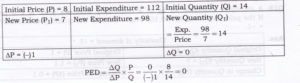## Question 11. A consumer demands 40 kg of a commodity when its price is Rs. 1 per kg. If the price increases by Rs. 0.10, what would be the quantity demanded? PED = -1.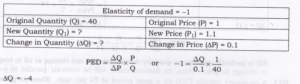## Question 12. PED = [-] 1. A consumer demands 50 units of a commodity when price is Rs 1 per unit. At what price will he demands 45 kg of a commodity?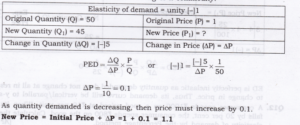## Question 13. A consumer spends Rs. 80 on a commodity when price is Rs 1 per unit. If the price increases by ?1, what would be his expenditure. PED = -0.4?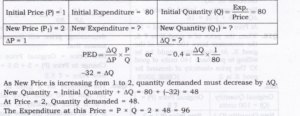## Question 14. The market demand for a good at Rs. 5 per unit is 50 units. Due to increase in price, the market demand falls to 30 units. Find out the new price if the price elasticity of demand is (-)2.